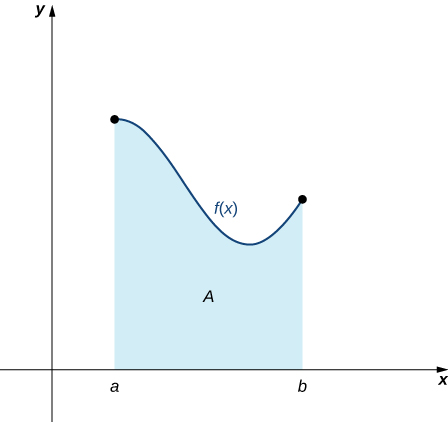# 5.1 Approximating areas  (Page 2/17)

 Page 2 / 17

Write in sigma notation and evaluate the sum of terms 2 i for $i=3,4,5,6.$

$\sum _{i=3}^{6}{2}^{i}={2}^{3}+{2}^{4}+{2}^{5}+{2}^{6}=120$

The properties associated with the summation process are given in the following rule.

## Rule: properties of sigma notation

Let ${a}_{1},{a}_{2}\text{,…,}\phantom{\rule{0.2em}{0ex}}{a}_{n}$ and ${b}_{1},{b}_{2}\text{,…,}\phantom{\rule{0.2em}{0ex}}{b}_{n}$ represent two sequences of terms and let c be a constant. The following properties hold for all positive integers n and for integers m , with $1\le m\le n.$

1. $\sum _{i=1}^{n}c=nc$

2. $\sum _{i=1}^{n}c{a}_{i}=c\sum _{i=1}^{n}{a}_{i}$

3. $\sum _{i=1}^{n}\left({a}_{i}+{b}_{i}\right)=\sum _{i=1}^{n}{a}_{i}+\sum _{i=1}^{n}{b}_{i}$

4. $\sum _{i=1}^{n}\left({a}_{i}-{b}_{i}\right)=\sum _{i=1}^{n}{a}_{i}-\sum _{i=1}^{n}{b}_{i}$

5. $\sum _{i=1}^{n}{a}_{i}=\sum _{i=1}^{m}{a}_{i}+\sum _{i=m+1}^{n}{a}_{i}$

## Proof

We prove properties 2. and 3. here, and leave proof of the other properties to the Exercises.

2. We have

$\begin{array}{cc}\sum _{i=1}^{n}c{a}_{i}\hfill & =c{a}_{1}+c{a}_{2}+c{a}_{3}+\text{⋯}+c{a}_{n}\hfill \\ & =c\left({a}_{1}+{a}_{2}+{a}_{3}+\text{⋯}+{a}_{n}\right)\hfill \\ \\ \\ & =c\sum _{i=1}^{n}{a}_{i}.\hfill \end{array}$

3. We have

$\begin{array}{cc}\sum _{i=1}^{n}\left({a}_{i}+{b}_{i}\right)\hfill & =\left({a}_{1}+{b}_{1}\right)+\left({a}_{2}+{b}_{2}\right)+\left({a}_{3}+{b}_{3}\right)+\text{⋯}+\left({a}_{n}+{b}_{n}\right)\hfill \\ & =\left({a}_{1}+{a}_{2}+{a}_{3}+\text{⋯}+{a}_{n}\right)+\left({b}_{1}+{b}_{2}+{b}_{3}+\text{⋯}+{b}_{n}\right)\hfill \\ \\ \\ & =\sum _{i=1}^{n}{a}_{i}+\sum _{i=1}^{n}{b}_{i}.\hfill \end{array}$

A few more formulas for frequently found functions simplify the summation process further. These are shown in the next rule, for sums and powers of integers , and we use them in the next set of examples.

## Rule: sums and powers of integers

1. The sum of n integers is given by
$\sum _{i=1}^{n}i=1+2+\text{⋯}+n=\frac{n\left(n+1\right)}{2}.$
2. The sum of consecutive integers squared is given by
$\sum _{i=1}^{n}{i}^{2}={1}^{2}+{2}^{2}+\text{⋯}+{n}^{2}=\frac{n\left(n+1\right)\left(2n+1\right)}{6}.$
3. The sum of consecutive integers cubed is given by
$\sum _{i=1}^{n}{i}^{3}={1}^{3}+{2}^{3}+\text{⋯}+{n}^{3}=\frac{{n}^{2}{\left(n+1\right)}^{2}}{4}.$

## Evaluation using sigma notation

Write using sigma notation and evaluate:

1. The sum of the terms ${\left(i-3\right)}^{2}$ for $i=1,2\text{,…,}\phantom{\rule{0.2em}{0ex}}200.$
2. The sum of the terms $\left({i}^{3}-{i}^{2}\right)$ for $i=1,2,3,4,5,6.$
1. Multiplying out ${\left(i-3\right)}^{2},$ we can break the expression into three terms.
$\begin{array}{cc}\sum _{i=1}^{200}{\left(i-3\right)}^{2}\hfill & =\sum _{i=1}^{200}\left({i}^{2}-6i+9\right)\hfill \\ \\ \\ & =\sum _{i=1}^{200}{i}^{2}-\sum _{i=1}^{200}6i+\sum _{i=1}^{200}9\hfill \\ & =\sum _{i=1}^{200}{i}^{2}-6\sum _{i=1}^{200}i+\sum _{i=1}^{200}9\hfill \\ & =\frac{200\left(200+1\right)\left(400+1\right)}{6}-6\left[\frac{200\left(200+1\right)}{2}\right]+9\left(200\right)\hfill \\ & =2,686,700-120,600+1800\hfill \\ & =2,567,900\hfill \end{array}$
2. Use sigma notation property iv. and the rules for the sum of squared terms and the sum of cubed terms.
$\begin{array}{cc}\sum _{i=1}^{6}\left({i}^{3}-{i}^{2}\right)\hfill & =\sum _{i=1}^{6}{i}^{3}-\sum _{i=1}^{6}{i}^{2}\hfill \\ \\ \\ \\ & =\frac{{6}^{2}{\left(6+1\right)}^{2}}{4}-\frac{6\left(6+1\right)\left(2\left(6\right)+1\right)}{6}\hfill \\ & =\frac{1764}{4}-\frac{546}{6}\hfill \\ & =350\hfill \end{array}$

Find the sum of the values of $4+3i$ for $i=1,2\text{,…,}\phantom{\rule{0.2em}{0ex}}100.$

15,550

## Finding the sum of the function values

Find the sum of the values of $f\left(x\right)={x}^{3}$ over the integers $1,2,3\text{,…,}\phantom{\rule{0.2em}{0ex}}10.$

Using the formula, we have

$\begin{array}{cc}\sum _{i=0}^{10}{i}^{3}\hfill & =\frac{{\left(10\right)}^{2}{\left(10+1\right)}^{2}}{4}\hfill \\ \\ & =\frac{100\left(121\right)}{4}\hfill \\ & =3025.\hfill \end{array}$

Evaluate the sum indicated by the notation $\sum _{k=1}^{20}\left(2k+1\right).$

440

## Approximating area

Now that we have the necessary notation, we return to the problem at hand: approximating the area under a curve. Let $f\left(x\right)$ be a continuous, nonnegative function defined on the closed interval $\left[a,b\right].$ We want to approximate the area A bounded by $f\left(x\right)$ above, the x -axis below, the line $x=a$ on the left, and the line $x=b$ on the right ( [link] ).An area (shaded region) bounded by the curve f ( x ) at top, the x -axis at bottom, the line x = a to the left, and the line x = b at right.

How do we approximate the area under this curve? The approach is a geometric one. By dividing a region into many small shapes that have known area formulas, we can sum these areas and obtain a reasonable estimate of the true area. We begin by dividing the interval $\left[a,b\right]$ into n subintervals of equal width, $\frac{b-a}{n}.$ We do this by selecting equally spaced points ${x}_{0},{x}_{1},{x}_{2}\text{,…,}\phantom{\rule{0.2em}{0ex}}{x}_{n}$ with ${x}_{0}=a,{x}_{n}=b,$ and

${x}_{i}-{x}_{i-1}=\frac{b-a}{n}$

for $i=1,2,3\text{,…,}\phantom{\rule{0.2em}{0ex}}n.$

We denote the width of each subinterval with the notation Δ x , so $\text{Δ}x=\frac{b-a}{n}$ and

${x}_{i}={x}_{0}+i\text{Δ}x$

A Function F(X)=Sinx+cosx is odd or even?
neither
David
Neither
Lovuyiso
f(x)=1/1+x^2 |=[-3,1]
apa itu?
fauzi
determine the area of the region enclosed by x²+y=1,2x-y+4=0
Hi
MP
Hi too
Vic
hello please anyone with calculus PDF should share
Which kind of pdf do you want bro?
Aftab
hi
Abdul
can I get calculus in pdf
Abdul
How to use it to slove fraction
Hello please can someone tell me the meaning of this group all about, yes I know is calculus group but yet nothing is showing up
Shodipo
You have downloaded the aplication Calculus Volume 1, tackling about lessons for (mostly) college freshmen, Calculus 1: Differential, and this group I think aims to let concerns and questions from students who want to clarify something about the subject. Well, this is what I guess so.
Jean
Im not in college but this will still help
nothing
how can we scatch a parabola graph
Ok
Endalkachew
how can I solve differentiation?
with the help of different formulas and Rules. we use formulas according to given condition or according to questions
CALCULUS
For example any questions...
CALCULUS
what is the procedures in solving number 1?
review of funtion role?
for the function f(x)={x^2-7x+104 x<=7 7x+55 x>7' does limx7 f(x) exist?
find dy÷dx (y^2+2 sec)^2=4(x+1)^2
Integral of e^x/(1+e^2x)tan^-1 (e^x)
why might we use the shell method instead of slicing
fg[[(45)]]²+45⅓x²=100
find the values of c such that the graph of f(x)=x^4+2x^3+cx^2+2x+2
anyone to explain some basic in calculus
I can
DebdootBy OpenStaxBy Joanna SmithbackBy Rachel CarlisleBy Hannah ShethBy Dravida Mahadeo-J...By OpenStaxBy Nick SwainBy OpenStaxBy OpenStaxBy Janet Forrester